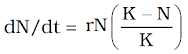# 13. ORGANISMS AND POPULATIONS

POPULATION GROWTH
The population size changes depending on factors like food availability, predation pressure & weather.

Changes in population density give some idea about the population – whether it is flourishing or declining.

4 basic processes that fluctuate the population density:
1. Natality (B): It is the number of births in a population during a given period.
2. Mortality (D): It is the number of deaths in a population during a given period.
3. Immigration (I): It is the number of individuals of the same species that have come into the habitat from elsewhere during a given time period.
4. Emigration (E): It is the number of individuals of the population who left the habitat and gone elsewhere during a given time period.
Natality & immigration increase the population density and mortality & emigration decrease the population density.

If N is the population density at time t, then its density at time t +1 is

Nt+1 = Nt + [(B + I) – (D + E)]

Population density increases if B+I is more than D+E. Otherwise it will decrease.

Under normal conditions, births & deaths are important factors influencing population density. Other 2 factors have importance only under special conditions. E.g. for a new colonizing habitat, immigration may be more significant to population growth than birth rates.

Growth Models

a. Exponential growth

Resources (food & space) are essential for the unimpeded population growth.

If resources are unlimited, each species shows its full innate potential to grow in number. Then the population grows in an exponential or geometric fashion.

If population size = N, birth rates (per capita births) = b and death rates (per capita deaths) = d, then the increase or decrease in N during a unit time period t (dN/dt) will be

dN/dt = (b – d) × N

Let (b–d) = r, then

dN/dt = rN

The r (‘intrinsic rate of natural increase’) is an important parameter for assessing impacts of any biotic or abiotic factor on population growth.

r value for the Norway rat = 0.015

r value for the flour beetle = 0.12

r value for human population in India (1981) = 0.0205

The integral form of the exponential growth equation is

Nt = Population density after time t

N0 = Population density at time zero

r = intrinsic rate of natural increase

e = the base of natural logarithms (2.71828)

Population growth curves

a = exponential growth (J-shaped curve)
b = logistic growth (Sigmoid curve)

b. Logistic growth

There is no population in nature having unlimited resources for exponential growth. This leads to competition among individuals for limited resources.

Eventually, the ‘fittest’ individuals survive and reproduce.

In nature, a given habitat has enough resources to support a maximum possible number, beyond which no further growth is possible. It is called carrying capacity (K).

A population with limited resources shows initially a lag phase, phases of acceleration & deceleration and finally an asymptote. This type of population growth is called Verhulst-Pearl Logistic Growth. It is described by following equation:Where N = Population density at time t

r = Intrinsic rate of natural increase

K = Carrying capacity

Since resources for growth for most animal populations are limited, the logistic growth model is more realistic.

Life History Variation

Populations evolve to maximise their reproductive fitness or Darwinian fitness (high r value). Under a particular set of selection pressures, organisms evolve towards the most efficient reproductive strategy.

Some organisms breed only once in their lifetime (Pacific salmon fish, bamboo) while others breed many times (most birds and mammals).

Some produce a large number of small-sized offspring (Oysters, pelagic fishes) while others produce a small number of large-sized offspring (birds, mammals).

These facts indicate that life history traits of organisms have evolved due to limited abiotic and biotic components of the habitat.
===========
👇 Select Your Topic Here 👇